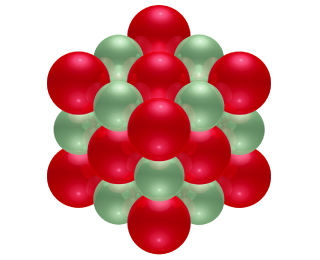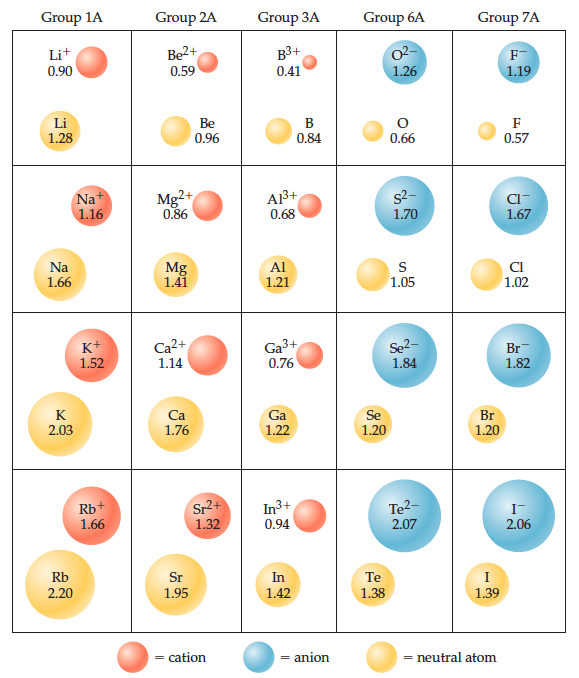# Problem: The ionic substance strontium oxide, SrO, forms from the direct reaction of strontium metal with molecular oxygen. The arrangement of the ions in solid SrO is analogous to that in solid NaCl and is shown here.The experimental density of SrO is 5.10 g/cm3. Based on the ionic radii in the figure below, predict the length of the side of the cube in the figure. Given your answer, what is the number of formula units of SrO that are contained in the cube in the figure?

90% (69 ratings)
###### Problem Details

The ionic substance strontium oxide, SrO, forms from the direct reaction of strontium metal with molecular oxygen. The arrangement of the ions in solid SrO is analogous to that in solid NaCl and is shown here.The experimental density of SrO is 5.10 g/cm3. Based on the ionic radii in the figure below, predict the length of the side of the cube in the figure. Given your answer, what is the number of formula units of SrO that are contained in the cube in the figure?Frequently Asked Questions

What scientific concept do you need to know in order to solve this problem?

Our tutors have indicated that to solve this problem you will need to apply the Unit Cell concept. You can view video lessons to learn Unit Cell. Or if you need more Unit Cell practice, you can also practice Unit Cell practice problems.